# C Program for Printing Next Successive and Adjacent Characters

In this tutorial, we will learn how to create a program in C that will print the next successive character and an adjacent character. First, we have created a program that will ask the user to enter any character to find and print its next successive character. Also, we have created a program that will take a character as input (from the user at run-time) and then print its adjacent character.

## C Print the Next Character

Let's first create a program that will print the next successive character of a given character entered by the user. Let's suppose that if the user has entered a as a character input, then the program will print b (the next successive character of a). Or, if the user types in the letter C, the program will print out the letter D.

The question is: write a program in C to accept a character from the user and process it in these two ways:

1. If the character is an alphabet, print the character after it. For example, if the user enters a, then print b. If x, then print y, and if z, then print a.
2. If it is not an alphabet, then print the character itself as output.

The answer to this question is given below:

```#include<stdio.h>
#include<conio.h>
int main()
{
char ch;
printf("Enter any character: ");
scanf("%c", &ch);
printf("\n");
if(ch>=65 && ch<90)
printf("%c", ch+1);
else if(ch>=97 && ch<122)
printf("%c", ch+1);
else if(ch==90)
printf("%c", 65);
else if(ch==122)
printf("%c", 122);
else
printf("%c", ch);
getch();
return 0;
}```

As the program given above was written in the Code::Blocks IDE, here is the sample run after a successful build and run: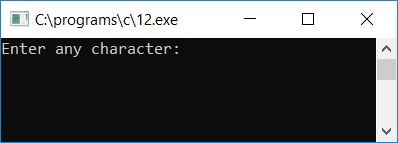Now supply any character as input, say "a," and press the ENTER key to see the next character that comes after "a." Here is the second snapshot of the sample run: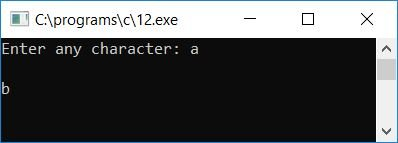Let's run another sample of the same program. This time, provide the input as Z and press the ENTER key: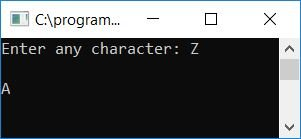Here is another sample run that checks what will happen if the user supplies any input that is not an alphabet character: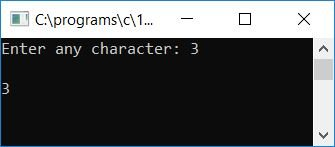#### Program Explained

• Receive any character as input.
• A-Z's ASCII code is 65-90. That works out to 65 for A, 66 for B, 67 for C, and 90 for Z.
• The ASCII code for letters a-z is 97-122. 97 for a, 98 for b, 99 for c,... 122 for z.
• Now check whether the character's ASCII code is greater than or equal to 65 and less than 90 or not.
• If it is, then increment the value of the character and print it out as the next successive character.
• Otherwise, check whether the character's ASCII code is greater than or equal to 97 and less than 122 or not.
• If it is, then again increment and print the value of the character as output.
• Here we have excluded the ASCII code for Z (capital) and z (small), because if the user enters z, then we have to print a as its successive character, and if the user enters Z, then we have to print A as its successive character, therefore we have checked using the ASCII code and printed the value accordingly.
• Finally, if none of the preceding conditions evaluate to true, print the character as is. That is, if the given character does not fall under a-z or A-Z, print the character as it is on the output screen.

## Print the adjacent characters of a given character in C

Let's create another program that will print the adjacent character of any given character entered by the user at run-time. In this case, adjacent characters are the two characters that come before and after the given character. For example, if the user has entered A, then print its adjacent characters as Z and B. Or if the user has entered D, then print C and E.

```#include<stdio.h>
#include<conio.h>
int main()
{
char ch;
printf("Enter any character: ");
scanf("%c", &ch);
printf("\n");
if(ch>65 && ch<90)
printf("Adjacent characters = %c and %c", ch-1, ch+1);
else if(ch>97 && ch<122)
printf("Adjacent characters = %c and %c", ch-1, ch+1);
else if(ch==90)
printf("Adjacent characters = %c and %c", ch-1, 65);
else if(ch==122)
printf("Adjacent characters = %c and %c", ch-1, 97);
else if(ch==65)
printf("Adjacent characters = %c and %c", 90, ch+1);
else if(ch==97)
printf("Adjacent characters = %c and %c", 122, ch+1);
else
printf("%c", ch);
getch();
return 0;
}```

Here is the final snapshot of the sample run: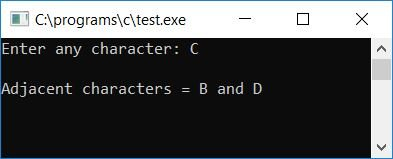The concept used in the preceding program is similar to that used in the preceding program. Except that we have to print the previous character along with the next character. Therefore, we have used ASCII code for A, a, Z, and z in separate cases.

C Quiz

« Previous Program Next Program »